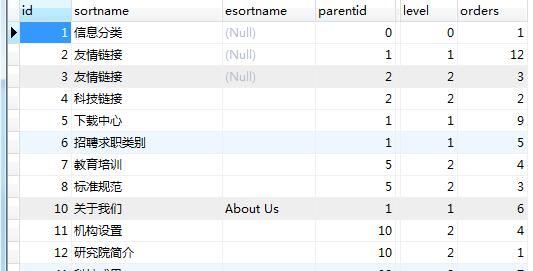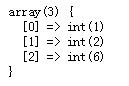## PHP引用及递归得到无限分类类别的所有父级类

1. 数据库表如下:2. 要得到一个类别的所有父类

```//得到文件所有的父级[数据读取使用的TP方便]
function getParentPath(\$id,\$table,&\$arr=[]){
\$pid = \think\Db::table(\$table)->where("id","=",\$id)->value("parentid");
if(\$pid){
\$arr[] = \$pid;
// dump(\$arr);
getParentPath(\$pid,\$table,\$arr);
}
return array_reverse(\$arr);
}```

3. 调用方便及显示结果

```\$table = "yt_areaclass";
\$path = getParentPath1(10,\$table);
dump(\$path);
```